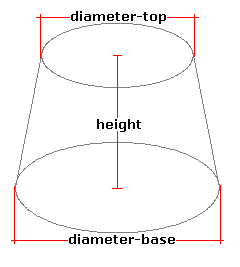Spike's Calculators

# Truncated Cone Volume and Area

Calculate the volume and the area of a truncated circular cone.This calculator works for any kind of measurement unit. For example, if you calculate in inches, inches will have to be used in all the length fields, and the area result will be square inches; the volume results in cubic inches and length measurements are in inches.

### Truncated Cone

Height of Cone #
Top Diameter #
Bottom Diameter #
Decimal Precision #

#### Results:

 Volume #³ Lateral Area #² Base Area #² Top Area #² Surface Area #² Slant Length # Slant Angle ° Surface to Volume Ratio sa:vol

#### Calculation

• the height of the cone
• top diameter of the cone
• bottom diameter of the cone
• decimal precision, number of digits after the decimal point

#### Results

• the volume of the cone volume (unit cubed)
• the lateral area of the cone (unit squared)
• the base (bottom) area of the cone (unit squared)
• the top area of the cone (unit squared)
• surface area of the cone (unit squared)
• slant length (starting unit)
• slant angle (degrees)
• surface area to volume ratio (decimal)
##### Formula
```V = 1/3Π((T/2)²+(T/2)(B/2)+(B/2)²)H;
LA = Π((T/2)+(B/2))√((T/2)-(B/2))²+H²
BA = Π(B/2)²
TA = Π(T/2)²
SL = √((B/2)-(T/2))²+H²
s = sin-1(H/SL)
LA  the lateral area of the truncuated cone
BA  the bottom area of the truncuated cone
TA  the top area of the truncuated cone
SL the length of the slant
s the angle of the slant
s/v = SA/V
where V is the volume of the truncuated cone
Π = 3.14159265
T the upper diameter
B the lower diameter
H the height of the cone
s/v is the surface area to volume ratio
```